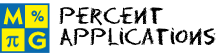# Percent ApplicationsThis page lists the Learning Objectives for all lessons in Unit 10.

#### Percent and Proportions

The student will be able to:

• Define percent, ratio proportion and equivalent fractions.
• Analyze percent problems to identify the given information and the unknown quantity.
• Assign a variable to represent the unknown quantity.
• Formulate a proportion to find the unknown quantity.
• Solve the proportion to find the unknown quantity.
• Explain the answer as it relates to the original problem statement.
• Recognize that the unknown quantity is different for each problem depending on how it is worded.
• Translate real-world problems into proportions involving percent.
• Apply percent and proportion concepts and procedures to complete five interactive exercises.

#### Discount and Sale Price

The student will be able to:

• Define percent, original price, rate, discount and sale price.
• Identify the rate from a real-world problem.
• Compute the discount.
• Compute the sale price.
• Describe the procedures for finding discount and sale price.
• Describe an alternate procedure for finding sale price and discount.
• Evaluate five interactive exercises with real-world problems.
• Analyze each problem to identify the given information.
• Formulate a strategy for solving each problem.
• Apply the procedures for finding discount and sale price to solve problems.

#### Simple Interest

The student will be able to:

• Define principal, interest, interest rate and time.
• Describe the formula for finding simple interest.
• Apply the formula for finding simple interest.
• Recognize the importance of converting time from months to years before applying the formula for interest.
• Recognize the difference between simple and compound interest.
• Compute simple interest.
• Evaluate five interactive exercises with real-world problems.
• Analyze each problem to identify the given information.
• Formulate a strategy for solving each problem.
• Apply the procedures for finding simple interest to solve problems.

#### Commission

The student will be able to:

• Define percentage, commission and straight commission.
• Identify the given information from real-world problems.
• Compute commission by applying percent concepts.
• Restate the formula for finding straight commission.
• Recognize that a commission can be earned or paid, depending on who is the seller and who is the client.
• Evaluate five interactive exercises with real-world problems.
• Analyze each problem to identify the given information.
• Formulate a strategy for solving each problem.
• Apply the procedure for finding commission to solve problems.

#### Sales Tax

The student will be able to:

• Define sales tax and tax rate.
• Describe how sales tax is collected.
• Compute sales tax by applying percent concepts.
• Describe the procedure for computing sales tax.
• Recognize that sales tax is included in the sale price in some countries.
• Evaluate five interactive exercises with real-world problems.
• Analyze each problem to identify the given information.
• Formulate a strategy for solving each problem.
• Apply sales tax procedures to solve problems.

#### Percent Change

The student will be able to:

• Define percent increase, percent decrease, and percent change.
• Examine how percent increase is computed in a real-world problem.
• Examine how percent decrease is computed in a real-world problem.
• Restate the formula for finding percent change.
• Identify the given information from real-world problems.
• Compute percent change from real-world problems.
• Distinguish between percent increase and percent decrease.
• Recognize that percent increase and percent decrease are measures of percent change.
• Evaluate five interactive exercises with real-world problems.
• Analyze each problem to identify the given information.
• Formulate a strategy for solving each problem.
• Apply percent change formulas and procedures to solve problems.

#### Practice Exercises

The student will be able to:

• Examine ten interactive exercises for all topics in this unit.
• Determine which formulas and procedures are needed to complete each practice exercise.
• Compute answers by applying appropriate formulas and procedures.
• Self-assess knowledge and skills acquired from this unit.

#### Challenge Exercises

The student will be able to:

• Evaluate ten interactive exercises with real-world problems for all topics in this unit.
• Analyze each problem to identify the given information.
• Formulate a strategy for solving each problem.
• Apply strategies to solve routine and non-routine problems.
• Synthesize all information presented in this unit.
• Connect percent applications to the real world.
• Develop problem-solving skills.

#### Solutions

The student will be able to:

• Examine the solution for each exercise presented in this unit.
• Identify which solutions need to be reviewed.
• Compare solutions to completed exercises.
• Identify and evaluate incorrect answers.
• Amend and label original answers.
• Identify areas of strength and weakness.
• Decide which formulas and procedures need to be reviewed from this unit.

### Order your CD today for Home or SchoolOR